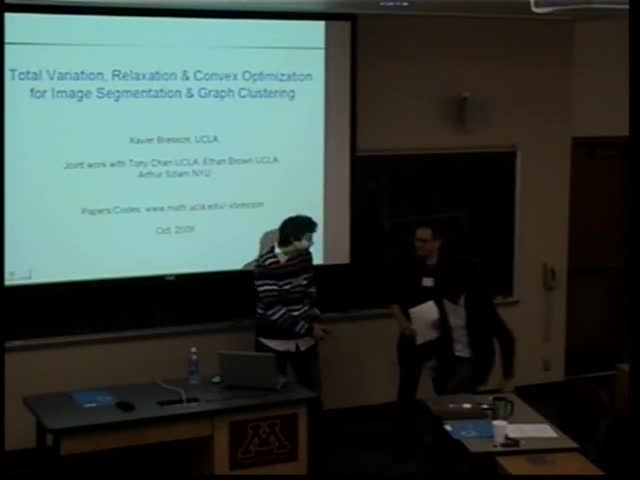## Total variation, relaxation & convex optimization for image segmentation & graph clustering

October 5, 2009
IMA
Presenters: Xavier Bresson

Watch Video

#### Abstract

Keywords: Image segmentation, Mumford-Shah model, graph clustering, relaxation method, total variation, operator splitting technique, normalized cut, Cheeger cut.

Abstract: In this talk, I will introduce two algorithms for image segmentation and graph clustering. One of the most influential image segmentation models is the Mumford-Shah’s model. Several algorithms such as the level set method have been introduced to compute a minimizing solution to the MS’s problem, but none of them can compute a global solution. We introduce a convex formulation for the multiphase piecewise constant MS problem (which is equivalent to the NP-hard problem of Potts in the discrete literature) and compute exact global minimizing solutions. We believe our method is the first in the literature that can make this claim. The second model will focus on graph clustering, which aims at grouping similar high-dimensional data s.a. images. The main problem of graph clustering is to minimize a cut of a graph. Popular cuts are the normalized cut of Shi-Malik and the Cheeger’s cut, which are NP-hard problems. We introduce a continuous relaxation of the Cheeger’s cut problem and we show that the relaxation is actually equivalent to the original problem, which is not the case with the Shi-Malik’s relaxation. We also give an algorithm which is experimentally efficient on some clustering benchmarks since the algorithm can cluster 10,000 high-dimensional points in a few seconds.

This is joint work with T.F. Chan, E. Brown (UCLA) and A. Szlam (NYU).